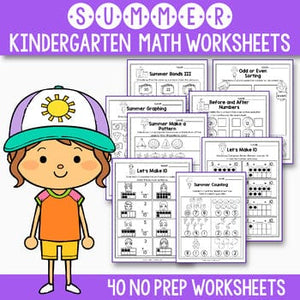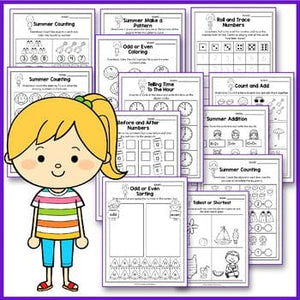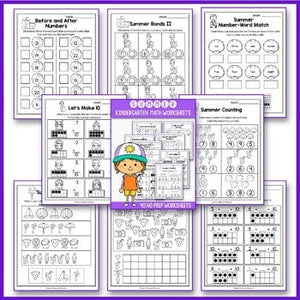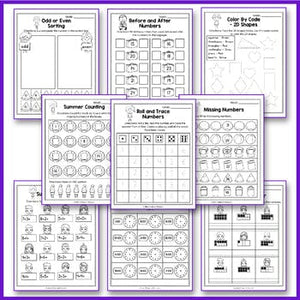# Summer Math Worksheet, End of Year Activities Math, Summer Packet Kindergarten

Regular price
\$3.00
Sale price
\$3.00
Tax included.

Are you looking for some fun  No Prep Summer Math Review Worksheets for your students? This packet will save you time and will keep them engaged every step of the way.

This math packet meets Common Core Standards for Kindergarten.

INCLUDES:
- Printable PDF - Summer Math No Prep Set (40 worksheets, 43 pages, 8.5 x 11 in)
- Cover
- Summary
- 40 Worksheet Pages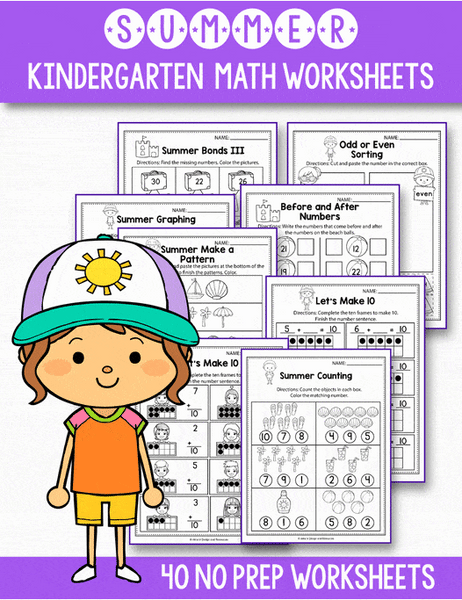WORKSHEETS INCLUDED:

• Summer Counting -count the summer pictures from each box and color the matching number
• Let's Make 10 - complete the ten frames to make 10. Finish the number sentence
• Adding With 10 - count the dots on the suitcases, solve the problems and write the results on the passports
• Summer Make A Pattern AB,AAB, ABC, AABB
• Match the Numbers - draw a line from a shell (tally marks) to its' matching number
• Match the Numbers  - draw a line from the luggage to its' matching ten base blocks
• Summer Graphing - create a graph using the pictures below
• Summer Graph and Tally
• Before and After Numbers (from 15 to 22) - write the numbers that come before and after the numbers on the beach balls
• Before and After Numbers (from 2 to 13) - write the numbers that come before and after the numbers on the clouds
• Summer More or Less (from 1 to 10) - compare each pair of numbers and write the correct equality sign in the middle
• Summer More or Less (from 11 to 20) - compare each pair of numbers and write the correct equality sign in the middle
• Summer More or Less (from 20 to 30) - compare each pair of numbers and write the correct equality sign in the middle
• Summer Bonds I (from 1 to 10) - find the missing numbers. Color the pictures
• Summer Bonds II (from 1 to 10) - find the missing numbers. Color the pictures
• Summer Bonds III (from 1 to 10) - find the missing numbers. Color the pictures
• Summer Number-Word Match - Cut And Glue - the ten frames to match them with the numbers on the shells
• Roll and Trace Numbers - roll a die, read the number and trace the number on its line
• Roll and Trace Numbers - roll a die, read the number and trace the number word on its line
• Missing Numbers (from 10 to 50) - write the missing numbers
• Summer Counting + Make an ABC pattern - count the shells by 1's.
• Summer Skip Count by 2's - write the missing numbers on each passport
• Summer Skip Count by 5's - write the missing numbers on each cloud
• Summer Skip Count by 2's - write the missing numbers on each beach ball
• Write the Number (from 1 to 10) - count the dots in each ten frame and write the numbers
• Summer Ten Frame (from 11 to 20)- fill in the frames to match the numbers next to them
• Summer Addition (from 1 to 10) - solve the problems and write the results
• Count and Add (from 1 to 10) - count the items in each box and add them together
• Summer Subtraction - count the objects in each box. Use the pictures to complete the equations
• Odd or Even Coloring (from 1 to 20) - color the odd numbers blue and the even numbers pink
• Color By Code - 2D Shapes - trace the 2D shapes, use the code to color the 2D shapes
• Telling Time To The Hour - draw the hands on the clock to match the digital clock
• Telling Time To The Hour - look at the clocks and write the time on the digital clocks
• Tallest or Shortest - in each box, color the shorter objects red and the taller objects green
• Before and After Numbers (from 2 to 13)
• Before and After Numbers (from 15 to 25)
• Odd Or Even Sorting